### Home > MC2 > Chapter 5 > Lesson 5.2.1 > Problem5-49

5-49.
1. Desmond is rolling a standard six-sided number cube. He plans to roll it 72 times. 5-49 HW eTool (CPM) Homework Help ✎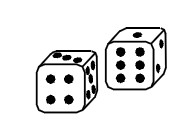1. About how many times would you expect Desmond to roll a 4? Why?

2. About how many times would you expect him to roll an even number? Why?

3. Desmond kept track of his results for all 72 rolls. The chart below shows some of his results.

Result

Number of outcomes

1

9

2

14

3

11

4

8

Based on his partial results, how many times did he roll a 5 or a 6?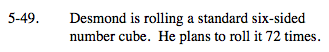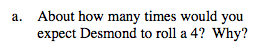On a six sided dice, if six possible outcomes are equally likely, what is the probability that you will roll a 4?

If you multiplied this probability by the total number of rolls, 72, how many rolls would you expect Desmond to roll a 4?

Be sure to know why this is the answer.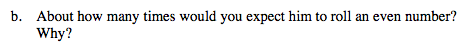On a six sided dice, half of the numbers:
2, 4, and 6 are even.

If three numbers out of six are even, what would the probability of rolling an even number be?
Multiply this number by the 72 rolls to find the expected number of rolls that would be even.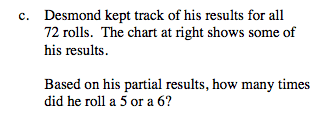If the total number of rolls is 72, and 42 of the rolls resulted in the numbers 1-4, how many times must Desmond have rolled 5 or 6?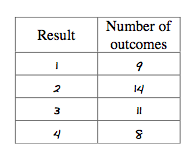30 times

Explore the problem with the eTool below.
Click the link at right for the full version of the eTool: MC2 5-49 HW eTool